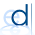## Wednesday, 26 June 2013

### IMAGE FILE TO TEXT FILE CONVERSION MATLAB – TEXT FILE TO IMAGE FILE CONVERSION MATLAB EXAMPLE

Here is an example to convert an image into a text file in matlab, for processing in HDL (verilog) or in someother tool. Also the text file is further converted into image file.

An image is a 2D matrix. For converting into text file, it needs to be converted into 1D matrix and then to text file using file write in matlab. The image size is also a constraint, so it needs to be fixed for conversion. Then converted to 1D matrix using reshape command. Then write into text file.

B=rgb2gray(A);
%figure,imshow(B),title('org');

C=imresize(B,[isize isize]);
figure,imshow(C),title('croped');
d=reshape(C,1,[]);
disp('Reshapping done');
fid = fopen('img.txt', 'wt');
fprintf(fid, '%8d\n', d);
disp('Text file write done');disp(‘ ‘);
fclose(fid);

For converting text file into image, it needs to be converted from 1D matrix and then to image. The image size must same as previous for processing text into image. Then converted to 1D matrix using reshape command. Then write into text file.

·         First, the text file is read using file read  as vector (1D matrix).
·         Then it has to be converted to matrix.
·         Finally transpose to complete it as image.

fidh = fopen('img.txt');
Ah = fscanf(fidh, '%g %g', [1 inf]);
fclose(fidh);
S1h= vec2mat(Ah,isize,isize);
disp('Vector conversion done');
%c=imresize(S1,[128 128]);
Sh= transpose(S1h);
Jh=uint8(Sh);
imwrite(Jh,['newimage','.jpg']);
figure,imshow(Jh),title('IMAGE form TEXT file');

OUTPUT:

If you have any queries mail us to
It helps us to do better.

Thank you.

1.how to easily read this file into verilog project?

1.thank u for ur comments , and ur request will be posted soon

2.This comment has been removed by the author.

3.This is very efficient as the ISE doesn't need to wait the OS to complete file read operation.

4.the text file generated after conversion is in hexadecimal or decimal number system?

5.can we get image to txt file in binary data, currently it is giving in decimal format? if yes then please let us know

1.@suguresh kumar :> yes it is possible. Convert the unsigned 8bit number to BINARY & write it to text file. Plz note to do it in loop for each element of 2D array.

6.its showing error as

Error using imwrite (line 459)
Can't open file "newimage.jpg" for writing.
You may not have write permission.

Error in image_file_io (line 31)
imwrite(Jh,['newimage','.jpg']);

7.Undefined function or variable 'isize'.